# How to Validate Data using express-validator Module in Node.js ?

• Difficulty Level : Expert
• Last Updated : 05 May, 2021

Validation in node.js can be easily done by using the express-validator module. This module is popular for data validation. There are other modules available in market like hapi/joi, etc but express-validator is widely used and popular among them.
Steps to install express-validator module:

1. You can install this package by using this command.

`npm install express-validator`
1. After installation, you can check your express-validator module version in command prompt using the command.

`npm version express-validator`
1. After that, you can just create a simple data as shown below to send the data to the server.
Filename: SampleForm.ejs

## html

 `` `<``html``>` `    ``<``head``>` `        ``<``title``>Validation using Express-Validator` `    ``` `<``body``>` `<``h1``>Demo Form` ` `  `<``form` `action``=``"saveData"` `method``=``"POST"``>` `  ``<``pre``>` `      ``Enter your Email    : <``input` `type``=``"text"` `name``=``"email"``> <``br``>` `      ``Enter your Name     : <``input` `type``=``"text"` `name``=``"name"``> <``br``>` `      ``Enter your Number   : <``input` `type``=``"number"` `name``=``"mobile"``> <``br``>` `      ``Enter your Password : <``input` `type``=``"password"` `name``=``"password"``> <``br``>` `      ``<``input` `type``=``"submit"` `value``=``"Submit Form"``> ` `  ``` `` ` `  `` ``

1. After that, you can just create a file, for example index.js as show below:
Filename: index.js

## javascript

 `const { check, validationResult }` `    ``= require(``'express-validator'``);`   `const bodyparser = require(``'body-parser'``)` `const express = require(``"express"``)` `const path = require(``'path'``)` `const app = express()`   `var` `PORT = process.env.port || 3000`   `// View Engine Setup` `app.set(``"views"``, path.join(__dirname))` `app.set(``"view engine"``, ``"ejs"``)`   `// Body-parser middleware` `app.use(bodyparser.urlencoded({ extended: ``false` `}))` `app.use(bodyparser.json())`   `app.get(``"/"``, ``function` `(req, res) {` `    ``res.render(``"SampleForm"``);` `})`   `// check() is a middleware used to validate` `// the incoming data as per the fields` `app.post(``'/saveData'``, [` `    ``check(``'email'``, ``'Email length should be 10 to 30 characters'``)` `                    ``.isEmail().isLength({ min: 10, max: 30 }),` `    ``check(``'name'``, ``'Name length should be 10 to 20 characters'``)` `                    ``.isLength({ min: 10, max: 20 }),` `    ``check(``'mobile'``, ``'Mobile number should contains 10 digits'``)` `                    ``.isLength({ min: 10, max: 10 }),` `    ``check(``'password'``, ``'Password length should be 8 to 10 characters'``)` `                    ``.isLength({ min: 8, max: 10 })` `], (req, res) => {`   `    ``// validationResult function checks whether` `    ``// any occurs or not and return an object` `    ``const errors = validationResult(req);`   `    ``// If some error occurs, then this` `    ``// block of code will run` `    ``if` `(!errors.isEmpty()) {` `        ``res.json(errors)` `    ``}`   `    ``// If no error occurs, then this` `    ``// block of code will run` `    ``else` `{` `        ``res.send(``"Successfully validated"``)` `    ``}` `});`   `app.listen(PORT, ``function` `(error) {` `    ``if` `(error) ``throw` `error` `    ``console.log(``"Server created Successfully on PORT "``, PORT)` `})`

Steps to run the program:

1. The project structure will look as shown below: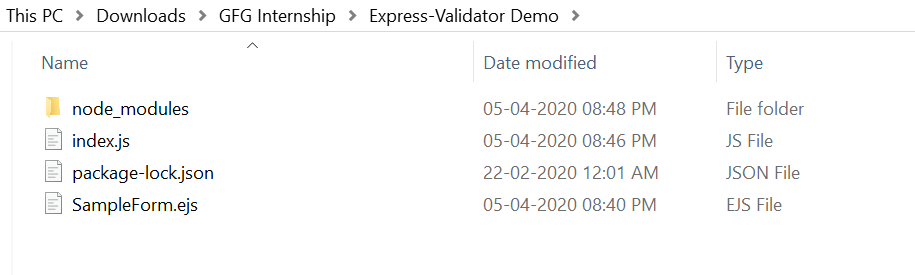1. Make sure you have a ‘view engine’. We have used “ejs” and also install express and express-validator, body-parser using following commands:

`npm install ejs`
1.
`npm install express`
1.
`npm install body-parser`
1.
`npm install express-validator`
1. Run index.js file using below command:

`node index.js`
1.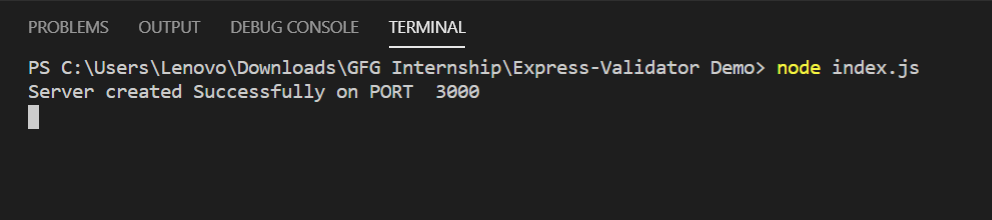1. Open the browser and type this URL http://localhost:8080/, the fill this sample form with correct data as shown below: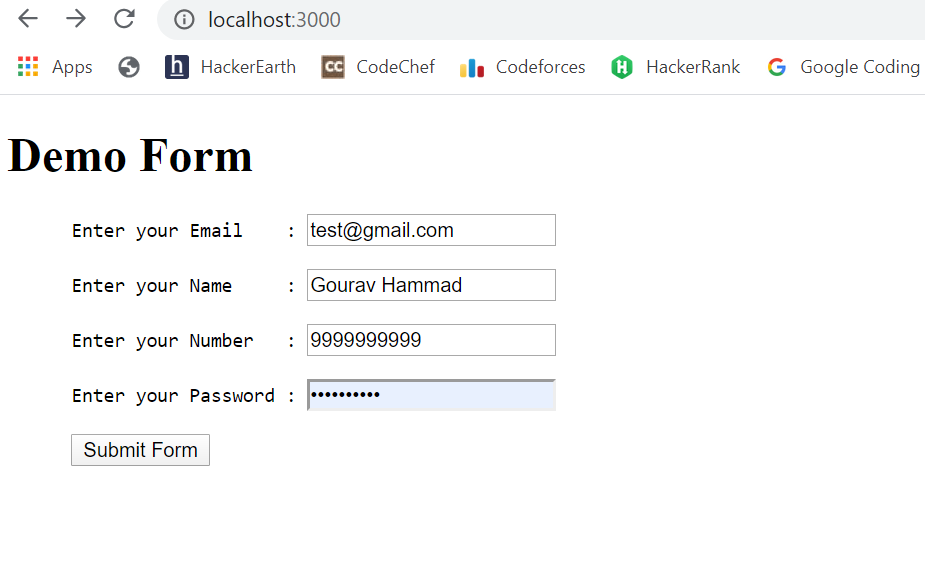1. Then submit the form and if no error occurs, then you will see the following output: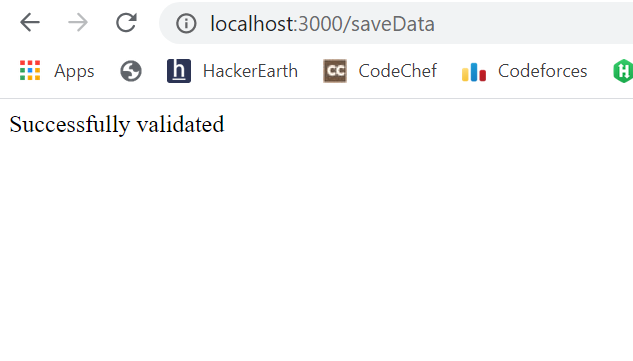1. And if you try to submit the form with incorrect data, then you will see the error message as shown below: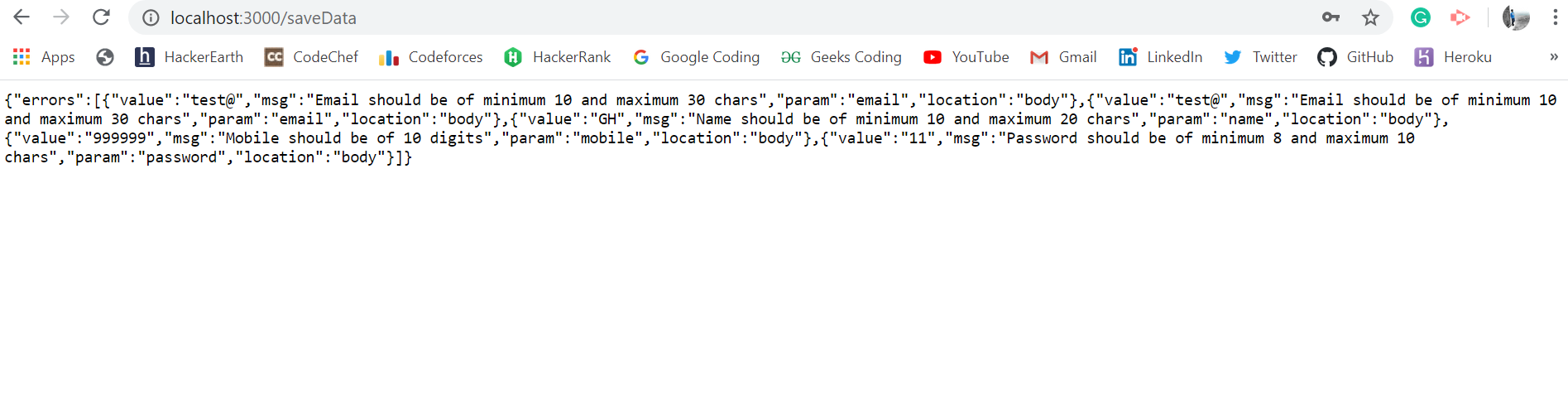1.

My Personal Notes arrow_drop_up
Recommended Articles
Page :